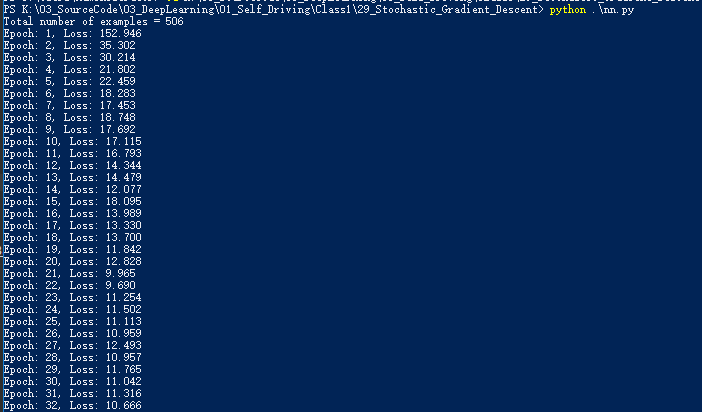# 随机梯度下降

1. 从总数据中随机选取一批数据
2. 使用步骤1的数据运行网络的forward和backward函数，计算梯度
3. 运行梯度下降更新方法
4. 重复步骤1-3

x=x−α∗(∂cost/∂x)

# 代码实现

``````def sgd_update(trainables, learning_rate=1e-2):
"""
Updates the value of each trainable with SGD.

Arguments:

`trainables`: A list of `Input` Nodes representing weights/biases.
`learning_rate`: The learning rate.
"""
# Performs SGD
#
# Loop over the trainables
for t in trainables:
# Change the trainable's value by subtracting the learning rate
# multiplied by the partial of the cost with respect to this
# trainable.
t.value -= learning_rate * partial  #参考上面的梯度下降更新公式
``````

SGD实现方式：

``````for i in range(epochs):
loss = 0
for j in range(steps_per_epoch):
# Step 1
# Randomly sample a batch of examples
X_batch, y_batch = resample(X_, y_, n_samples=batch_size)

# Reset value of X and y Inputs
X.value = X_batch
y.value = y_batch

# Step 2
forward_and_backward(graph)

# Step 3
sgd_update(trainables)

loss += graph[-1].value
``````

sklearn.utils.resampleGithub链接